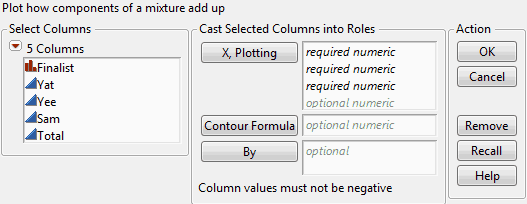Essential Graphing > Ternary Plots > Launch the Ternary Plot Platform
Publication date: 08/13/2020

# Launch the Ternary Plot Platform

Launch Ternary Plot by selecting Graph > Ternary Plot.

Figure 11.5 The Ternary Plot Launch WindowX, Plotting

Assign three columns to generate a ternary plot.

If you assign more than 3 variables to the X, Plotting role, a matrix of ternary plots is created. A separate variable is assigned to the first two axes of a plot, with the third axis being the sum of the other variables. If necessary, the variables are scaled so they sum to 1.

Contour Formula

To plot contours of a response surface, assign a column containing a formula to the Contour Formula role. If you have variables in a Contour formula that are not listed as X, Plotting variables, JMP appends sliders below the plot so that the values can be interactively adjusted. See Example Using a Contour Function.

By

This option produces a separate graph for each level of the By variable.

After you click OK, the Ternary Plot window appears. See The Ternary Plot.